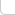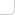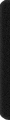# 某岛

… : "…アッカリ～ン . .. . " .. .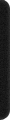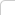July 26, 2021

# 传送门

https://codeforces.com/contest/1552

# Problem C.n <= 10

# Problem E. Colors and Intervals

(n, k <= 100)???

# Problem F. Telepanting

```a[i] += x[i]-y[i]; REP(j, i) if (x[j] >= y[i]) a[i] += a[j];```

# Problem I. Organizing a Music Festival

(n <= 100)

（我傻了，这不是 PQ 树么。。。）